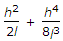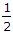# Civil Engineering - Surveying - Discussion

Discussion Forum : Surveying - Section 1 (Q.No. 2)
2.
If h is the difference in level between end points separated by l, then the slope correction is. The second term may be neglected if the value of h in a 20 m distance is less thanm
1 m
2 m
3 m
Explanation:
No answer description is available. Let's discuss.
Discussion:
66 comments Page 1 of 7.

Logeshkanna said:   3 years ago
The answer should be A. 1/2m.

With ref of Punmia : the second term in slope correction shall be neglected for slopes flatter than about 1 in 25 (i.e slope should be lesser than 0.04).

The slope is Vertical distance/Horizontal distance.
.
Here Vertical distance is h and the horizondal distance is √((L)^2 - (h)^2).

L = 20 given in the problem. We need to find, for which value of h the slope will be less than 0.04.

Sub h = 0.5, we get slope = 0.025;
Sub h = 1, we get slope = 0.050;
Sub h = 2, we get slope = 0.100;
Sub h = 3, we get slope = 0.150;

Therefore Option A (h=0.5m) is the correct answer.

Abhilash jana said:   8 years ago
When distances are measured along the slope, the equivalent horizontal distance may be determined by applying a slope correction.

The vertical slope angle of the length measured must be measured. (Refer to the figure on the other side).

Thus, For gentle slopes, m<20%.
Ch = h^2/2l.

For steep slopes, 20% <= m <= 30%.
Ch = h^2/2l+h^4/8l^3.

For very steep slopes, m>30%.
Ch = l(1-cos0).

Pravin Pawar said:   7 years ago
For slope greater than 5% closer a closer approximation of corrected slope can be determined by h2/2l + h4/8l3.
Otherwise taken as h2/2l.

In the above example slope taken as 5% of length.
Length = 20 m therefore slope is 1.
Slope = height/length.

We have slope value equal to 1.
Then h2/2l = 1.
Answer is 6.32 m which is more than 3.

Deepesh said:   7 years ago
Two things I found in this discussion,

1) For a slope smaller than 10%, Slope correction = h^2/2l, and,
2) For a slope greater than 10%, Slope correction = h^2/2l + h^4/8l^3 should use.

But finally, I did not get the answer 3 or 3.333.

VIVEK CHANDEL said:   9 years ago
For slopes of 10 percent or less, the correction to be applied to L for a difference d in elevation between tape ends, or for a horizontal offset d between tape ends, may be computed from,

Cs=(h^2)/(2L).

For a slope greater than 10 percent, Cs may be determined from,
Cs={(h^2)/(2L)}+{(h^4)/8(L^3)}.

Aman said:   9 years ago
If we use any value lesser than 3 and not greater than 3, then answer for slope correction either using the complete formula given above or by eliminating the second term, we will get the same answer. Thus it means that the second term can be eliminated the right answer is 3 and distance kept as 20m.

For slopes greater than 5% the above formula must be adopted otherwise the second part of the formula can be neglected.

Since the first three options are less than or equal to 5% they can neglected as the 3m is greater than 5% the second part of formula must included. Hence the answer is (D) 3m.

Frlyrvllz said:   4 years ago
The formula (h^2/2L)+(h^4/8L^3) is for slope that ranges from 20%-30%.

If (h^4/8L^3) is to be neglected, h^2/2L is left and if we are going to equate it with 20% the answer would be 2.8~3 and if h^2/2L is to be equated to 30% the answer would be 3.4~3 therefore the answer is 3.

Imran Hossain said:   7 years ago
** If h is the difference in level between endpoints separated by l, then the slope correction is c=(h^2/2l)+{h^4/8(l^3)}.

** If h is less than 3m in a length often the 20 m, then the quantity h^4/8(l^3) can be neglected. Hence c = h^2/2l.

Source. Dr. B.C. Punmia.

Fayez abusafaqa ps said:   3 years ago
The error can be accepted upto 10%. thus, the second term divided by the correlational factor equal 0.1.

Assuming the first term is x and the second term is y. Then y/x+y=0.1==> x+y/x=10 with y not zero==>1+x/y=9==>y=9. solve it u will get l=3.